Home > INT3 > Chapter 11 > Lesson 11.2.4 > Problem11-104

11-104.
1. Solve each equation for 0 ≤ x < 2π. No calculator should be necessary. Homework Help ✎

1.cos(x) = –2. tan(x) =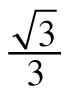3. sin(x) = 0

4. cos(x) =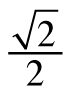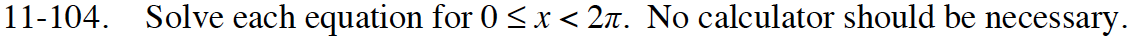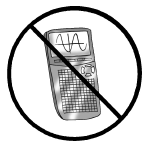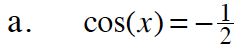$\text{Recall that } \cos \left( \frac{\pi}{6}\right) = \frac{1}{2}, \text{ so the reference angle will be 30°.}$

In which quadrants is cosine negative? Sketch a unit circle with right triangles with 30° angles at the origin in the second and third quadrants. What are the circular angles?

x = 120° and 240°

Now convert the angles into radians.

$120\left(\frac{\pi}{180}\right)\ \ \ \ \ \ \ \ 240\left(\frac{\pi}{180}\right)$

$x = \frac{2{\pi}}{3}, \frac{4{\pi}}{3}$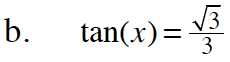See part (a).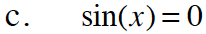If sin(x) = 0, x = 0.

x = 0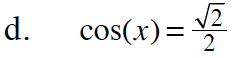Remember the side ratios of 45° right triangles.